# Nominal GDP Vs Real GDP Part II of

• Slides: 15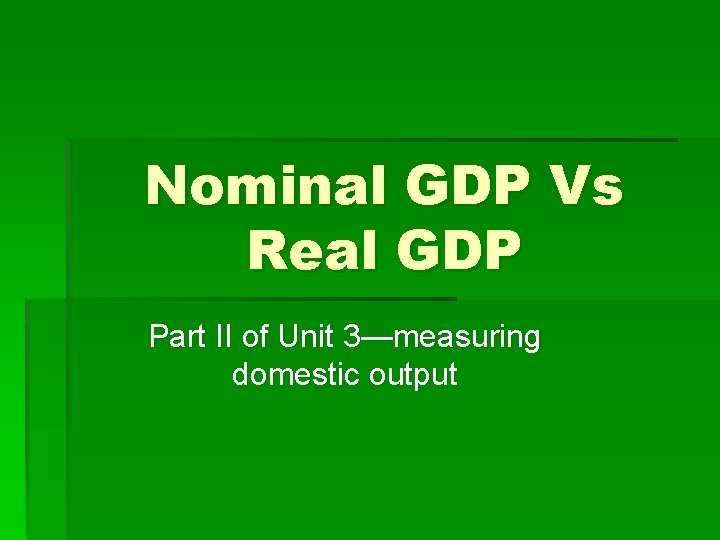Nominal GDP Vs Real GDP Part II of Unit 3—measuring domestic outputGDP § Reminder: GDP is a figure including every item produced in the economy. § Money is the common denominator that allows us to add the total output.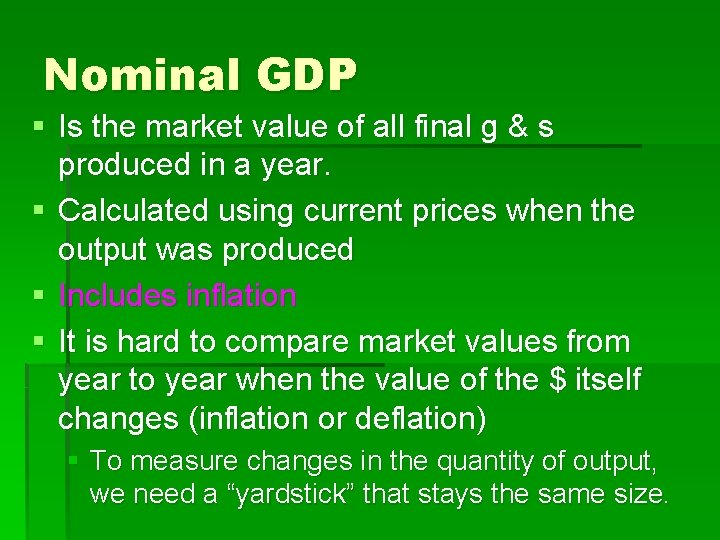Nominal GDP § Is the market value of all final g & s produced in a year. § Calculated using current prices when the output was produced § Includes inflation § It is hard to compare market values from year to year when the value of the \$ itself changes (inflation or deflation) § To measure changes in the quantity of output, we need a “yardstick” that stays the same size.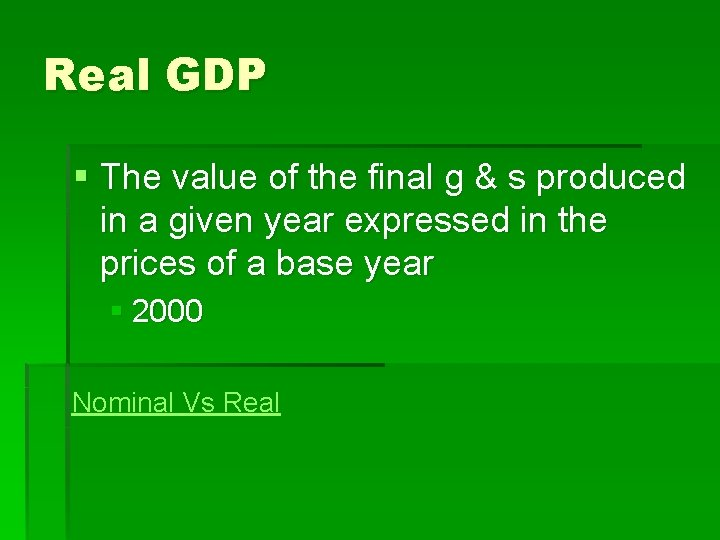Real GDP § The value of the final g & s produced in a given year expressed in the prices of a base year § 2000 Nominal Vs Real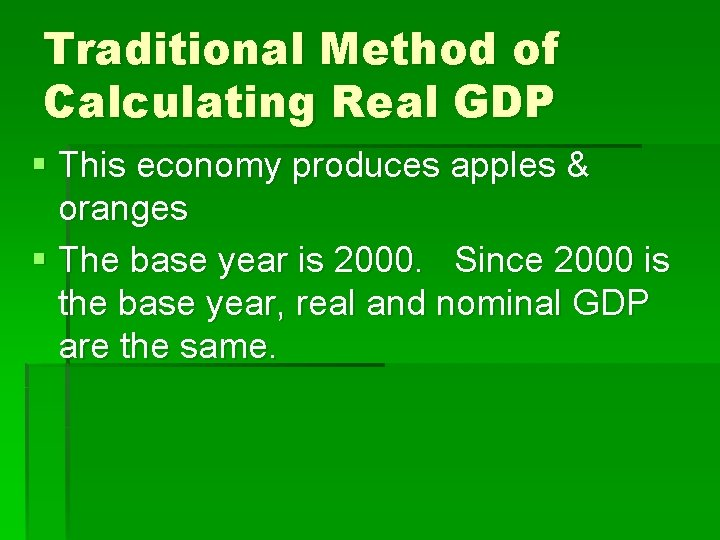Traditional Method of Calculating Real GDP § This economy produces apples & oranges § The base year is 2000. Since 2000 is the base year, real and nominal GDP are the same.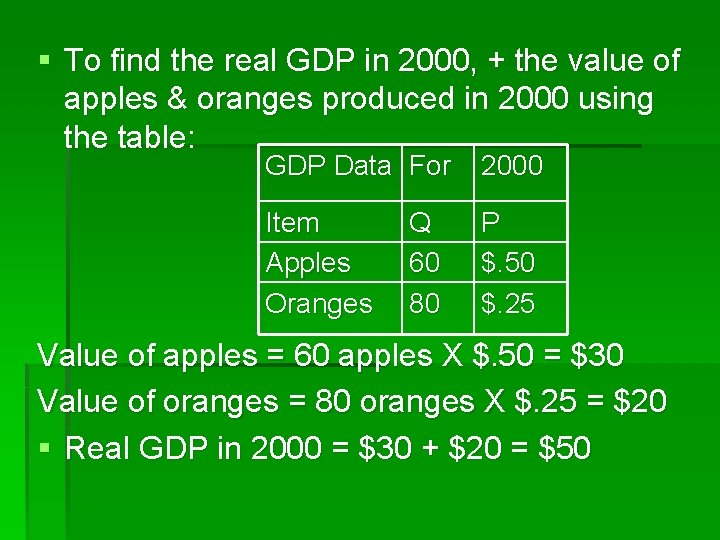§ To find the real GDP in 2000, + the value of apples & oranges produced in 2000 using the table: GDP Data For 2000 Item Apples Oranges P \$. 50 \$. 25 Q 60 80 Value of apples = 60 apples X \$. 50 = \$30 Value of oranges = 80 oranges X \$. 25 = \$20 § Real GDP in 2000 = \$30 + \$20 = \$50§ To calculate real GDP in 2006, + the value of apples and oranges using the prices of 2000 GDP Data Item Apples Oranges For Q 160 220 2006 P \$1. 00 \$2. 00 § Value of apples = 160 apples X \$. 50 = \$80 § Value of oranges = 220 oranges X \$. 25 = \$55 § Real GDP in 2006 = \$80 + \$55 = \$135 § Real GDP is “constant dollars” or “ 2000 dollars” measure (taken inflation out)2 purposes of estimating Real GDP § To compare the standard of living over time (based on quantity, not price) § To compare the standard of living among countriesPrice Index § A measure of the price of a specified collection of g & s (market basket) in a given year as compared to the price of an identical collection of g & s in a reference year. § PI = price of market basket for a specific year X 100 price of same market basket in the base year § Find Real GDP = Nominal GDP X 100 PI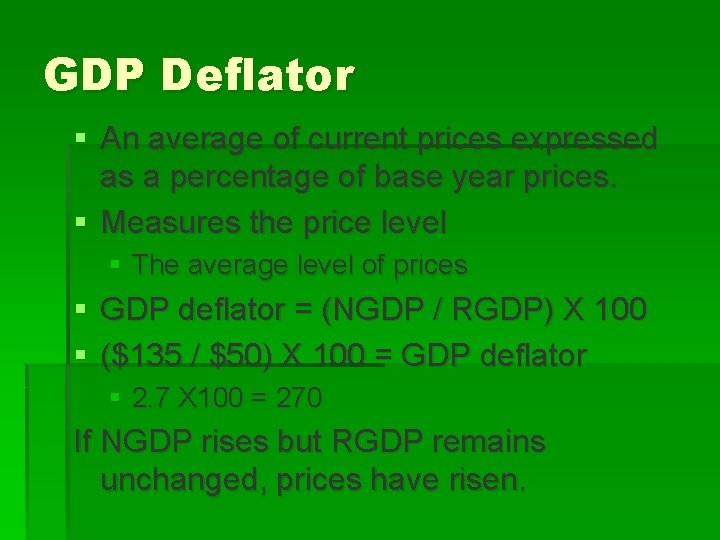GDP Deflator § An average of current prices expressed as a percentage of base year prices. § Measures the price level § The average level of prices § GDP deflator = (NGDP / RGDP) X 100 § (\$135 / \$50) X 100 = GDP deflator § 2. 7 X 100 = 270 If NGDP rises but RGDP remains unchanged, prices have risen.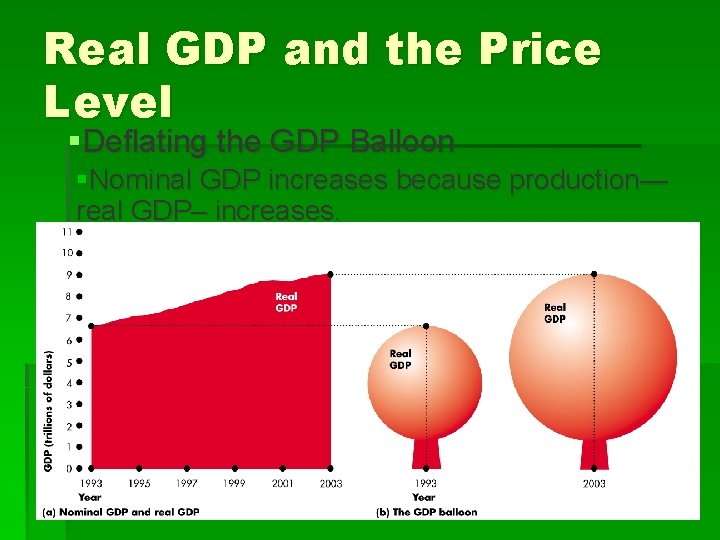Real GDP and the Price Level §Deflating the GDP Balloon §Nominal GDP increases because production— real GDP– increases.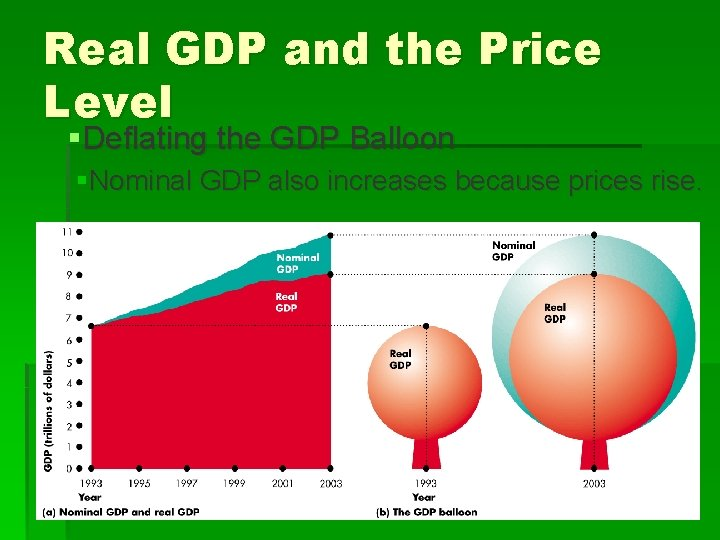Real GDP and the Price Level §Deflating the GDP Balloon §Nominal GDP also increases because prices rise.Real GDP and the Price Level §Deflating the GDP Balloon §We use the GDP deflator to let the air out of the nominal GDP balloon and reveal real GDP.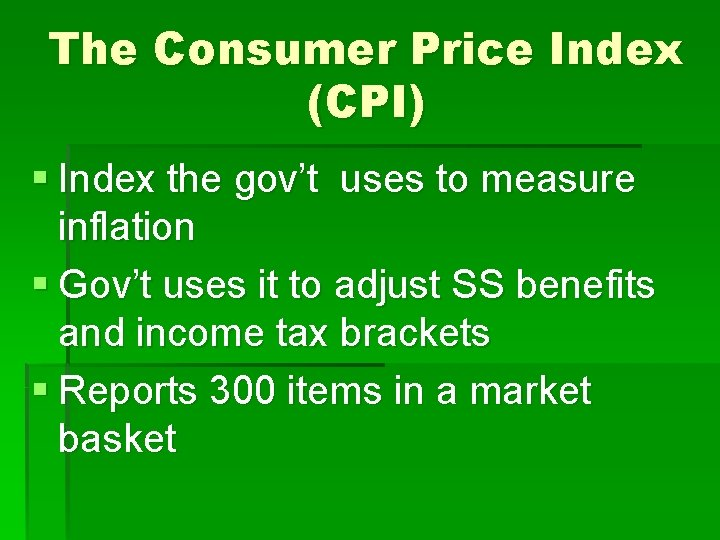The Consumer Price Index (CPI) § Index the gov’t uses to measure inflation § Gov’t uses it to adjust SS benefits and income tax brackets § Reports 300 items in a market basketInflation § A rise in the general level of prices § Inflation rate = current CPI-Index CPI = rate (X 100)= % index CPI or Year 2 – Year 1 = rate (X 100) = % Year 1